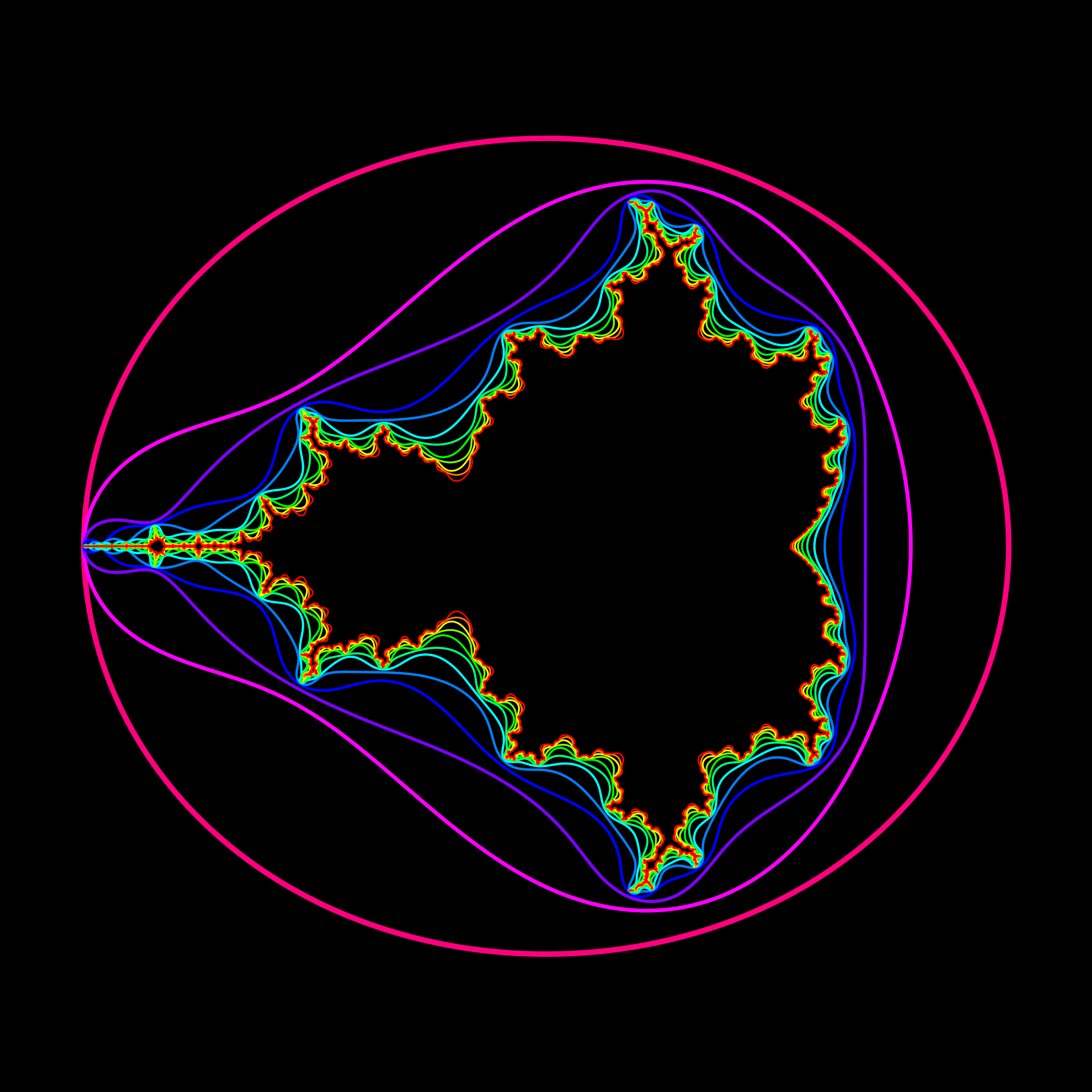# Monte Carlo integration

What is the area of the Mandelbrot set? We do not have an analytic expression for it. But we can try to approximate it numerically. One way to do that is Monte Carlo Integration.

The Mandelbrot set is the set of complex numbers M = { c = a+i b | Tc(0)n stays ; bounded where Tc(z) =z2+c. In real coordinates the map is Tc(x,y) = (x2-y2+a,2xy + b). The notation Tn means applying the map T a number of times. For example T3(x,y) = T(T(T(x,y))). One can draw numerically the level curves Tn(x,y) = 2 as if we outside the region, then we are outside the Mandelbrot set. here is some code to compute the area of the Mandelbrot set using Monte Carlo computation.
```M=Compile[{x,y},Module[{z=x+I y,k=0},
While[Abs[z]<2.&&k<999,z=N[z^2+x+I y];++k];Floor[k/999]]];
n=10^6; 9*Sum[M[-2+3*Random[],-1.5+3*Random[]],{n}]/n
```
There is a lot of discussion about the area in Example. This paper gives a value of 1.506793 with pixel counting. Google gives 1.506484, with a 95% confidence interval from 1.506480 to 1.506488.